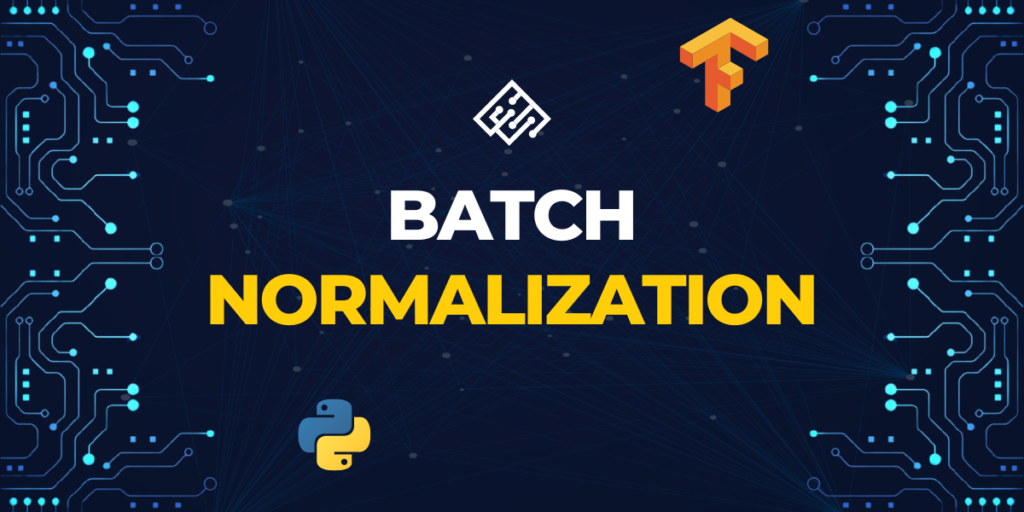# Batch Normalization for Deep Neural NetworksBefore delving into the topic, let’s know more about what “normalization” is. When the features in the data have different ranges, normalization is an approach used during data processing, to adjust the values of numeric columns in a dataset to a similar scale.

There are different types of normalization:

• Batch Normalization
• Layer Normalization
• Instance Normalization
• Group Normalization

In this article, we will learn about batch normalization, the difference between normalization and batch normalization, what is the need of batch normalization and what are the benefits of performing batch normalization. Here’s a medium article that talks about the subject in more detail.

Batch normalization is a method for training deep neural networks that normalizes the contributions to a layer for each mini-batch. This has the effect of stabilizing the learning process and significantly reducing the number of training epochs needed to build deep neural networks

## How is normalization different from batch normalization?

In deep learning, before passing data to the neural network, pre-processing is done. Some techniques include standardization and normalization. Both have the same objective of transforming the data and putting all the data on the same scale. In standardization, the mean is subtracted from the point and is then divided by the standard deviation. While in normalization, the given range of values is normalized to a new range.

Usually, this range is set to [0,1] as this range will ensure that the problem of exploding gradient won’t occur. Batch normalization consists of normalizing activation vectors from hidden layers using the mean and variance of the current batch. This normalization step is applied right before (or right after) the nonlinear activation function. This process is done in batches hence the name batch normalization.

In layman’s terms, normalization is applied at the input, before passing the data to the network whereas batch normalization happens inside the network, within hidden layers.

## What is the need for batch normalization?

Now that we have normalized the data at the prior stage, we have tackled the exploding gradient problem and the training speed is increased relatively. It’s been observed that, even after this normalization, models tend to be slow and unstable. What could be the reason behind it? Let’s discuss this in brief.

We know how neural networks learn. In order for neural networks to learn, weights associated with neuron connections must be updated after the forward passes of data through the network. In most of cases, stochastic gradient descent is followed, so what if one of the weights ends up becoming drastically larger than others.

This will then cause output from its corresponding neuron to be extremely large and this imbalance will again continue to cascade through the neural network causing instability.

This is where batch normalization comes into the picture. The batch norm is applied to layers that you choose to apply it to within your network. when applying batch norm to a layer the first thing the batch norm does is normalize the output from the activation function

## Implementing Batch Normalization

Since, we are scaling and shifting the inputs, there are two associated variables corresponding to each of these. These are learnable parameters.

We can see epsilon in the normalization equation. This is to make sure that the variance is never zero. After updating each Xi’s, batch will have 0 mean and some standard deviation. But mean and std deviation will highly be dependent on the batch samples. i.e., for every batch, mean and variance should be different.

Thus, two learnable parameters gamma and beta are used. After training number of batches, gamma should tend to actual mean and beta to actual std deviation.

Let’s see an example of a neural network where batch normalization is used:

```from keras.models import Sequential
from keras.layers import Dense, BatchNormalization

model = Sequential()
#This is the Batch normalization layer

```

You can also learn about building networks from scratch. In this example, the model is a simple feedforward neural network with two hidden dense layers and an output layer with a `softmax` activation function. After each dense layer, we added a `BatchNormalization` layer to normalize the activations. This can be done using the `BatchNormalization` class in Keras, which has several optional parameters such as `momentum`, `epsilon`, and `center`, which you can use to customize the behavior of the batch normalization layer. To know more about this, refer to the official documentation from tensorflow.

## Summary

Now that we have understood, why we do it and how to implement it; let us breifly discuss how it benefits the neural network.

• It reduces internal covariate shift.
• Increases speed of training
• Decreases the importance of initial weights
• Regularizes the mode